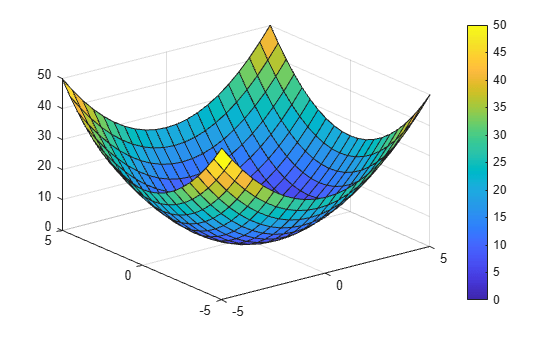# clim

Set colormap limits (Renamed from `caxis` in R2022a)

## Syntax

``clim(limits)``
``clim("auto")``
``clim("manual")``
``clim(target,___)``
``lims = clim``

## Description

example

````clim(limits)` sets the colormap limits for the current axes. `limits` is a two-element vector of the form ```[cmin cmax]```. All values in the colormap indexing array that are less than or equal to `cmin` map to the first row in the colormap. All values that are greater than or equal to `cmax` map to the last row in the colormap. All values between `cmin` and `cmax` map linearly to the intermediate rows of the colormap. NoteThe `clim` function only affects graphics objects that have the `CDataMapping` property set to `"scaled"`. It does not affect graphics objects that use truecolor or have the `CDataMapping` set to `"direct"`. ```
````clim("auto")` enables automatic limit updates when values in the colormap indexing array change. This is the default behavior. The ```clim auto``` command is an alternative form of this syntax.```

example

````clim("manual")` disables automatic limit updates. The ```clim manual``` command is an alternative form of this syntax.```

example

````clim(target,___)` sets the colormap limits for a specific axes or standalone visualization. Specify `target` as the first input argument in any of the previous syntaxes.```

example

````lims = clim` returns the current colormap limits as a vector of the form `[cmin cmax]`.```

## Examples

collapse all

Plot a paraboloid with a colorbar.

```[X,Y] = meshgrid(-5:.5:5); Z = X.^2 + Y.^2; surf(Z); colorbar```Get the current color limits.

`lims = clim`
```lims = 1×2 0 50 ```

Raise the lower limit to `20`. Notice that all values of `Z` that are less than or equal to `20` map to the first color.

`clim([20 50])`Create two paraboloid surfaces that are vertically offset.

```[X,Y] = meshgrid(-5:.5:5); Z1 = X.^2 + Y.^2; Z2 = Z1 + 50;```

Plot the first paraboloid. Add a colorbar, and hold the axes for the second paraboloid.

```surf(X,Y,Z1); colorbar hold on```Hold the current color limits using the "`manual"` option. Then plot the second paraboloid.

```clim("manual"); surf(X,Y,Z2);```Call the `tiledlayout` function to create a 1-by-2 tiled chart layout. Call the `nexttile` function to create the axes objects `ax1` and `ax2`. Plot a paraboloid into each axes.

```[X,Y] = meshgrid(-5:1:5); Z = X.^2 + Y.^2; tiledlayout(1,2) % Left plot ax1 = nexttile; surf(ax1,Z); % Right plot ax2 = nexttile; surf(ax2,Z);```Raise the lower color limit of the right axes to `20`.

`clim(ax2,[20 50])`## Input Arguments

collapse all

New limits, specified as a vector of the form `[cmin cmax]`. The value of `cmin` must be less than `cmax`.

Data Types: `single` | `double`

Target object, specified as an `Axes` object, `PolarAxes` object, a `GeographicAxes` object, or a standalone visualization that has a `Colormap` property.

collapse all

### Colormap Indexing Array

An array that maps data elements in a chart to specific rows in the colormap. MATLAB® stores the indexing array as a property on the graphics object.

For example, the `CData` property of a `Surface` object is an indexing array that maps grid points on the surface to specific rows in the colormap.

## Version History

Introduced before R2006a

expand all